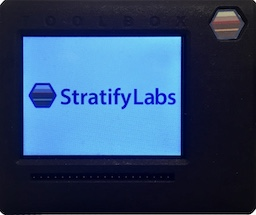# ADC Thermistor Circuit and Lookup Table###### Introducing the Stratify Toolbox

Are you a printf()-er or a code-stepper? The Stratify Toolbox is a printf()-ing awesome debug tool!Thermistors are simple to integrate into embedded designs but their temperature response can be challenging to interpret. A lookup table is a convenient way to convert a thermistor’s ADC reading to temperature.

A thermistor’s resistance varies with temperature. As an example, assume T1 in the circuit above to be part number NTCLE413E2103H400 from Vishay/Dale Inc. This thermistor has the resistive characteristics shown in the table below. At cold temperatures, the resistance is very large but decreases non-linearly as temperature increases.The complete datasheet for the part referenced is available here. The voltage at the ADC input is calculated using a voltage divider:

$$V_{ADC} = V_1 \frac{R_1}{R_1+T_1}$$

When the $$T_1$$ resistance is large, the voltage at the ADC input is close to zero. As the thermistor gets warmer, the voltage gets closer to $$V_1$$. The transition, however, is non-linear. This means for embedded firmware to interpret the ADC reading as a temperature, it needs to either calculate a complicated transfer function or approximate the temperature using a lookup table and linear extrapolation.

## Lookup Tables

A lookup table consists of two (or more) columns of data, in this case, a column for the voltage representing the output of the sensing circuit and a column for the temperature.

## Creating the Lookup Table

A spreadsheet program is an excellent tool to create (and update) the lookup table and can be designed to easily copy and paste as a data table in a C file. The image below shows a screenshot of an example. The highlighted portion can be directly copied and pasted into the code.The table contains distinct points on the voltage temperature curve. Linear extrapolation is used to estimate the temperature between the points.

## Extrapolating the Data

To extrapolate the data between points, the firmware first needs to select two data points. It must scan the values in the x-column and find the two values directly above and below the input. The firmware then uses the point-slope formula to extrapolate the temperature value.

$$m = \frac{y_0-y_1}{x_0-x_1}$$

$$y = m \cdot (x_0 - x_1) + y_1$$

The values x0, x1, y0, and y1 are taken from the lookup table. The x value is the input from the ADC, and the y value is the temperature. The code below implements a lookup table using floating-point variables.

#include <stdint.h>
#include <stdio.h>

typedef struct {
float x;
float y;
} dsp_lookup_f_t;

float dsp_lookup_f(const dsp_lookup_f_t * table, float x, int size){
int i;
float m;
i = 0;
while((i < (size)) &amp;&amp; (x > table[i].x) ){  //find the two points in the table to use
i++;
}
if ( i == size ){   //make sure the point isn't past the end of the table
return table[i-1].y;
}

if ( i == 0 ){  //make sure the point isn't before the beginning of the table
return table[i].y;
}

m = (table[i].y - table[i-1].y) / ( table[i].x - table[i-1].x); //calculate the slope
return m * (x - table[i].x) + table[i].y; //this is the solution to the point slope formula
}

#define TABLE_SIZE 17
//The table below is copied and pasted from the spreadsheet
dsp_lookup_f_t my_table[TABLE_SIZE] = {
{ .x=  11839  ,  .y=  -40  },
{ .x=  16050  ,  .y=  -35  },
{ .x=  21385  ,  .y=  -30  },
{ .x=  27980  ,  .y=  -25  },
{ .x=  35921  ,  .y=  -20  },
{ .x=  45214  ,  .y=  -15  },
{ .x=  55761  ,  .y=  -10  },
{ .x=  67358  ,  .y=  -5  },
{ .x=  79703  ,  .y=  0  },
{ .x=  92422  ,  .y=  5  },
{ .x=  105128  ,  .y=  10  },
{ .x=  117450  ,  .y=  15  },
{ .x=  129084  ,  .y=  20  },
{ .x=  139810  ,  .y=  25  },
{ .x=  149497  ,  .y=  30  },
{ .x=  158097  ,  .y=  35  },
{ .x=  165626  ,  .y=  40  },
}

float temp;